Next: Learning procedure Up: Optimising the cost function Previous: Updating the network weights   Contents

### Updating the hidden states

The basic setting for updating the hidden states is the same as above for the network weights. The correlations between consecutive states cause some changes to the formulas and require new ones for adaptation of the correlation coefficients. All the feedforward computations use the marginal variances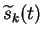which are not actual variational parameters. This affects the derivatives with respect to the other parameters of the state distribution. Let us use the notation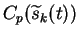to mean that the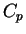part of the cost function is considered to be a function of the intermediate variables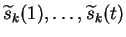in addition to the variational parameters. This and Equation (5.48) yield following rules for evaluating the derivatives of the true cost function: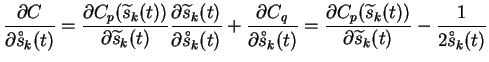(6.35)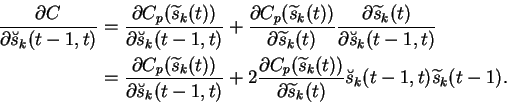(6.36)

The term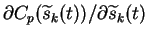in the above equations cannot be evaluated directly, but requires again the use of new intermediate variables. This leads to the recursive formula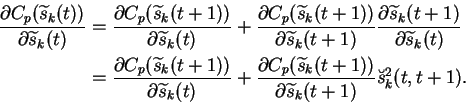(6.37)

The terms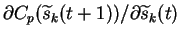are now the ones that can be evaluated with the backward computations through the MLPs as usual.

The term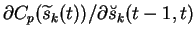is easy to evaluate from Equation (6.33), and it gives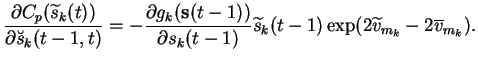(6.38)

Equations (6.38) and (6.40) yield a fixed point update rule for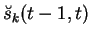: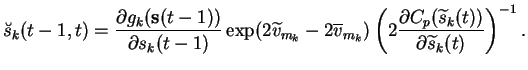(6.39)

The result depends, for instance, on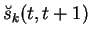through Equation (6.39), so the updates must be done in the order starting from the last and proceeding backward in time.

The fixed point update rule of the variances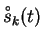can be solved from Equation (6.37):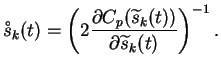(6.40)

The update rule for the means is similar to that of the weights in Equation (6.36) but it includes a correction which tries to compensate the simultaneous updates of the sources. The correction is explained in detail in .Next: Learning procedure Up: Optimising the cost function Previous: Updating the network weights   Contents
Antti Honkela 2001-05-30